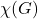# Graph Coloring ProblemA node coloring is valid or admissible if any two adjacent nodes do not have the same color. If a graph is colorable, there is a smallest number such that the graph is node colorable. This number is called the chromatic number of the graph and is usually denoted by.

The problem to identify the chromatic number of a graph is np-hard, meaning that from the complexity theory point of view there is probably no algorithm that solves the problem efficiently.

## Determine the Chromatic Number by Hand

At first, if you just want to find an admissible coloring of a graph , you can do that using a heuristic approach. A possible heuristic is the greedy one presented here at javatpoint.com:

Step 1: In the first step, we will color the first vertex with first color.

Step 2: Now, we will one by one consider all the remaining vertices (V -1) and do the following:

• We will color the currently picked vertex with the help of lowest number color if and only if the same color is not used to color any of its adjacent vertices.
• If its adjacent vertices are using it, then we will select the next least numbered color.
• If we have already used all the previous colors, then a new color will be used to fill or assign to the currently picked vertex.

Let’s apply this heuristic for the graph shown above:

Instead of applying colors, integer numbers starting at 1 have been assigned representing different colors. We start in the middlemost node, color it with color 1, then color all nodes on the “outer” subgraph with the lowest admissible integer (color), resulting in alternating colors 2 and 3. Then, we continue with the “inner” subgraph, assigning 1 to the left node and 2 to the lower node. Finally, we end up with the right node of the inner subgraph, where we cannot apply any color of 1, 2 or 3. We need a foruth color here.

No matter what heuristic you choose, you often cannot be sure, whether you really found the minimal number of colors. Whatever coloring heuristic you use, you probably won’t find one, which succeeds to find the minimal number of colors, i.e. the chromatic number, for any connected graph (if you do, please let me know :)).

For this reason, I want to present you a way, how you can find out, whether the number of colors you found really represent the graph’s chromatic number.

## Determine the Chromatic Number Constraint Programming

Another solution to solve problems like these is provided by constraint programming. MiniZinc is a free open source modelling language allowing you to model constraint satisfaction and optimization problems in a high-level, solver-independent way. The software provides a graphical IDE and comes with a set of different solvers. You can attach other constraint programming solvers like the award winning CP-SAT solver from google or-tools or even commercial MILP solvers like CPLEX or GUROBI.

Now what are the steps we need to take, to find out whether “our” chromatic number we found (i.e. 4) is correct for the given graph or not?

• define the presumed chromatic number
• transform our graph into a set of color variables for each node
• formulate the constraints to prevent equally colored neighbor nodes
• try to solve the model for satisfyability
• reduce the chromatic number by one and retry solving until unsatisfyable

The following script shows a possible transformation of the graph above into a cp-program using the MiniZinc IDE (download here):

int: nc = 4;       % max number of colors allowed

var 1..nc: n1;     % define color variable for node 1
var 1..nc: n2;     % define color variable for node 2
var 1..nc: n3;     % define color variable for node 3, ...
var 1..nc: n4;
var 1..nc: n5;
var 1..nc: n6;
var 1..nc: n7;
var 1..nc: n8;
var 1..nc: n9;
var 1..nc: n10;

constraint n1 != n2;     % color of node1 must not be equal to color of node2,
constraint n1 != n7;     % color of node1 must not be equal to color of node7, ...
constraint n1 != n6;
constraint n1 != n10;
constraint n2 != n10;
constraint n2 != n3;
constraint n3 != n10;
constraint n3 != n4;
constraint n3 != n8;
constraint n4 != n5;
constraint n4 != n10;
constraint n5 != n6;
constraint n5 != n9;
constraint n5 != n10;
constraint n6 != n10;
constraint n7 != n8;
constraint n7 != n9;
constraint n8 != n9;

solve satisfy;                  % solve model for satisfyability

output ["n1=\(n1)\t n2=\(n2)\t n3=\(n3)\n",       % plot results of variables
"n4=\(n4)\t n5=\(n5)\t n6=\(n6)\n",
"n7=\(n7)\t n8=\(n8)\t n9=\(n9)\n",
"n10=\(n10)\t \n"];

When we run this script in MiniZinc, we get the following output:

Running nodecoloring.mzn
n1=2	 n2=3	 n3=2
n4=3	 n5=2	 n6=3
n7=4	 n8=3	 n9=1
n10=1
----------
Finished in 221msec.

Now let’s try to find a solution with 3 colors, by setting variable nc = 3 and running the script.

Running nodecoloring.mzn
=====UNSATISFIABLE=====
Finished in 1s 407msec.


Now we know, that the graph really has the chromatic number of 4 and there is no admissible solution to color the graph with only 3 colors.

Categories: